# Noise, Turbulence, and Texture

Dr. Matthew O. Ward
Computer Science Department
WPI

Random Numbers in Graphics

• Random numbers can be used on all graphical attributes (location, color, normals, transparency, motion)

• Many natural phenomena are composed of a combination of structure and randomness

Just Call random()

• We can simply call random() with an appropriate value range and distribution

• This doesn't provide any continuity between adjacent points

• Can lead to "bubbling" effect when animated

Fields of Random Noise

• Generate a fixed grid of random numbers (1-D, 2-D, ....) of size N in each dimension

• Assuming a single continuous dimension in data space with variable T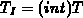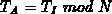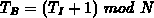• noise(T) is influenced by noise() and noise(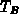)

Linear Interpolation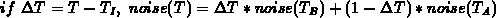This gives smooth transitions between nodes, but only 0th order continuity at nodes.

Easy to extend to arbitrary dimensions.

Polynomial Fits are Better

• Can fit quadratic or cubic curves to fill in between nodes (treat random number as a dimension)
• Can use nodes as control points for splines
• Can assign gradients at nodes and use Hermite curves

Here is an example of a random field using Catmull-Rom splines for interpolation.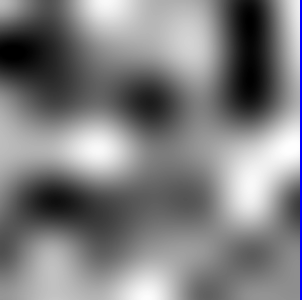Noise is Good, Turbulence is Better

We can impose self-similarity on noise to add scale-invariance. The turbulence at a point is created by summing the noise at that point with scaled down noise values at other points.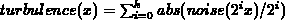where k is the smallest integer for which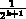is greater than the size of a pixel in real coordinates. This is very similar to fractal surface generation, giving a visual impression of brownian motion.

Here is an example of a turbulent field using the random field as a basis. I show it using grey scale and a random color map.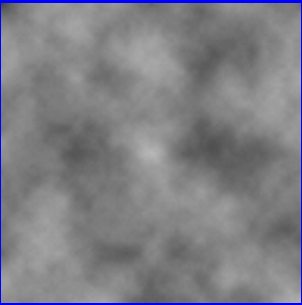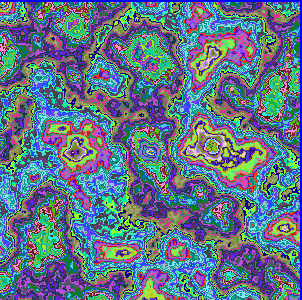Using Turbulence

2. Impose turbulence on values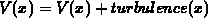or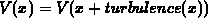3. Need to modify range of turbulence to fit structure

4. Need to play with degree and scale of turbulence

Applications

Marble Texture: Add turbulence to sine wave, use a curve through color space instead of a ramp

Here is an example of a sine wave with added turbulence. I show it using grey scale and a random color map.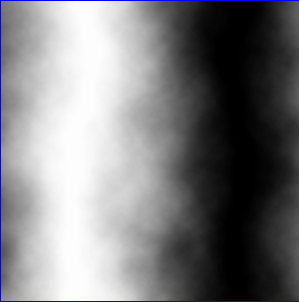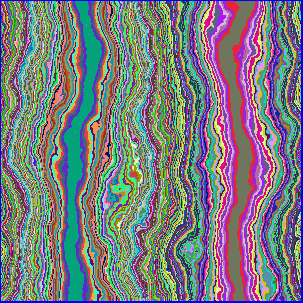Tree Bark: Add turbulence to a sawtooth wave and use to bump map surface

Stars: Intensity of points based on distance to the center. Add turbulence to this distance. Here is an example of a star with added turbulence.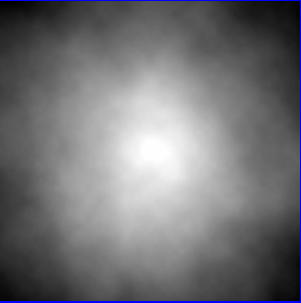Flames: Compute intensity of point based on distance from center in x. Scale it based on distance in y. Add turbulence. Use 3-D turbulence to animate. Here is an example of a flame with added turbulence.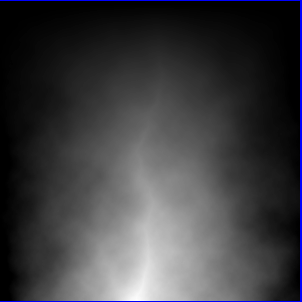Conclusions

• Lots of interesting effects can be gained by adding turbulence

• Need to play with degree and scale to get most realistic images

• Ties together a lot of topics in graphics (fractals, texture, color, curves)

matt@owl.WPI.EDU
Tue Apr 25 11:51:57 EDT 1995